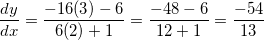# High School Math : Using Implicit Differentiation

## Example Questions

### Example Question #72 : Calculus I — Derivatives

An ellipse is represented by the following equation: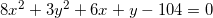What is the slope of the curve at the point (3,2)?

undefined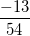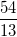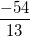Explanation:

It would be difficult to differentiate this equation by isolating. Luckily, we don't have to.  Use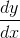to represent the derivative ofwith respect toand follow the chain rule.

(Remember,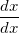is the derivative ofwith respect to, although it usually doesn't get written out because it is equal to 1. We'll write it out this time so you can see how implicit differentiation works.)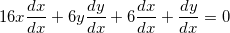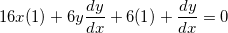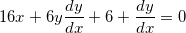Now we need to isolateby first putting all of these terms on the same side: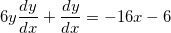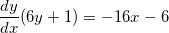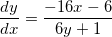This is the equation for the derivative at any point on the curve. By substituting in (3, 2) from the original question, we can find the slope at that particular point: